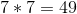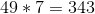# ISEE Upper Level Math : How to find the missing part of a list

## Example Questions

2 Next →

### Example Question #11 : Sets

Find the missing part of the list: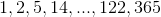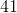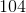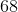Explanation:

The sequence pattern follows the rule: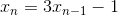Therefore,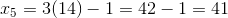.

### Example Question #12 : Sets

Which of the following can be the next number after 47 in the sequence below?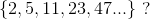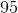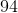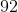Explanation:

Each number in the set is the value of the preceding number multiplied by 2, plus 1: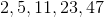Therefore, the next number in the set will be 47 times 2, plus 1. This gives us:### Example Question #13 : Sets

Jack noticed that a group of prime numbers followed a pattern in which they increased by 2, then 4, in an alternating pattern: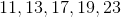(11 plus 2 is 13, 13 plus 4 is 17, 17 plus 2 is 19, 19 plus 4 is 23.)

Jack believes that this pattern applies for all prime numbers, not just this group.

Which of the following is the best counter argument to his belief?

19 is not a prime number.

Jack made a mistake on some of the addition in the set.

The next prime number, 29, breaks the pattern because it is 6 greater than 23.

7 is 4 less than 11.

The next prime number, 29, breaks the pattern because it is 6 greater than 23.

Explanation:

While Jack's pattern applies to the group of number that he selected, it will not apply for the next prime number, 29, because it is 6 greater than the previous prime number of 23. Therefore, the correct answer is:

The next prime number, 29, breaks the pattern because it is 6 greater than 23.

### Example Question #14 : Sets

Find the value of x.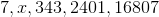Explanation:

Find the value of x.We have a geometric series here. In other words, each term is a consistent multiple of the number before it. To find our common multiple, we need to take one term and divide it by the term before it. Let's try using the 3rd and 4th terms.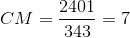So, to move from one term to the next, we need to multiply by seven.

To find x, we need to multiply our first term (7), by 7.